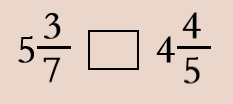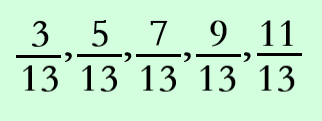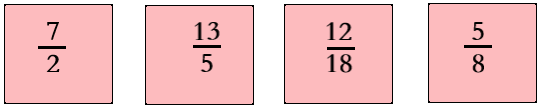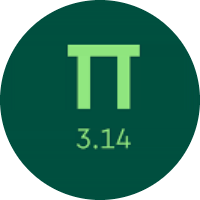# Learn the Concept of Fractions

In this quiz, you will get to know about the basics of fractions. You will find problems of equivalent fractions, writing fractions in lowest terms, improper, mixed fractions, comparing fractions, and putting fractions in order.

Start Quiz

20 students went to the zoo. 12 of them saw the penguins. Which fraction of students saw the penguins?

3/5

2/5

4/519/3

15/3

7/3

Which sign will make the statement true?<

=

>

What will be the next fraction in the series?1

14/13

1/2

15/13

Which benchmark is between 7/10 and 17/13?

1

1/2

What is the least common denominator of 3/5 and 4/7?

5

35

12

7

Put these fractions in order from least to greatest.##5/8## < ##12/18## < ##13/5## < ##7/2##

12/18 5/8 13/5 7/2

What is the reciprocal of 6/11?

11/6

6/5

Which fractions are equal to 3/5? Select all that apply.

9/15

10/25

12/20

12/15

Quiz/Test Summary
Title: Learn the Concept of Fractions
Questions: 9
Contributed by: